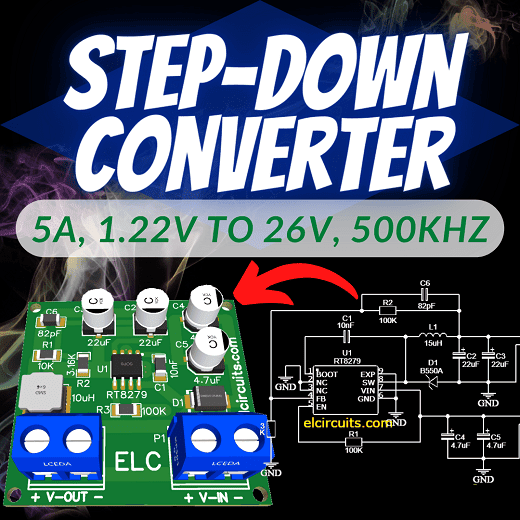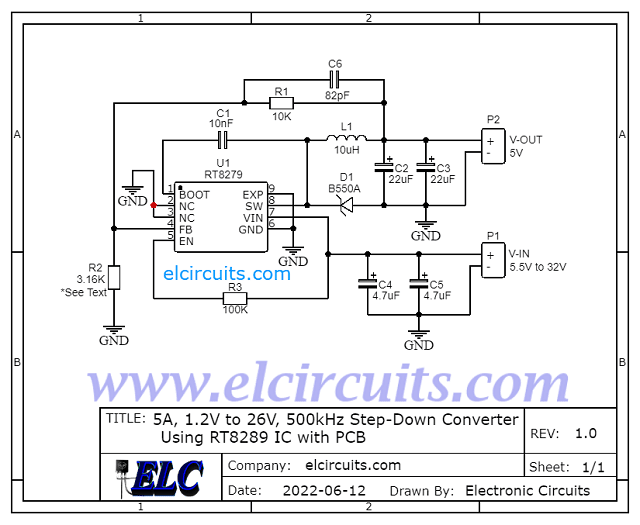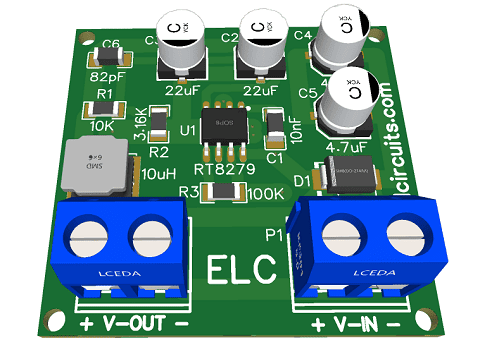## Wednesday, June 22, 2022Fig. 1 - 5A, 1.22V to 26V, 500kHz Step-Down Converter Using RT8289 IC with PCB

This is a DC-DC 5A, 1.22V to 26V, 500kHz Step-Down Converter Using RT8289 IC, it works with a Step-Down conversion system.

This powerful chip manages to work with very few external components and provides a preset voltage between 1.22V to 26V, with 5 Amps of output current.

## General  IC Description

The RT8289 is a step-down regulator with an internal Power MOSFET. It achieves 5A of continuous output current over a wide input supply range with excellent load and line regulation.

Current mode operation provides fast transient response and eases loop stabilization. The RT8289 provides protections such as cycle-by-cycle current limiting and thermal shutdown.

In shutdown mode, the regulator draws 25A of supply current. The  RT8289  requires  a  minimum  number  of  external components, to provide a compact solution. The  RT8289  is  available  in  a  SOP-8  (Exposed  Pad) package.

## Features

• High Output Current up to 5A
• Internal Soft-Start
• 100mΩ Internal Power MOSFET Switch
• Internal  Compensation  Minimizes  External  Parts Count
• High Efficiency up to 90%
• 25μA Shutdown Current
• Fixed 500kHz Frequency
• Thermal Shutdown Protection
• Cycle-by-Cycle Over Current Protection
• Wide 5.5V to 32V Operating Input Range
• Adjustable Output  Voltage from 1.222V to 26V
• Available in an SOP-8 (Exposed Pad) Package
• RoHS Compliant and Halogen Free

## Output Voltage Setting

To define the output voltage, we use a voltage divider formed by 2 resistors, R1 and R2, this allows the FB pin of the integrated circuit to detect changes in the output voltage, and recalibrate the circuit keeping it stabilized.

To set this output voltage, we can calculate the external resistive divider, according to the equation formulated below:

• VOUT = VREF *(1 + (R1/R2))
• Where VREF is the reference voltage (type 1.222V).
• Where R1 = 10kΩ.
We exemplify in our circuit, the voltage divider is formed by R1 and R2.

#### General Formula:

• VOUT = VREF *(1 + R1/R2)
• Where VREF is the reference voltage (type 1.222V).
• Where R1 = 10kΩ.

#### For a 3.3V output, our formula would look like:

• Vout = 1,222 * (1+ (10/5.8))
• Vout = 3.328V

#### For a 5V output, our formula would look like:

• Vout = 1,222 * (1+ (10/3.16))
• Vout = 5,089V

#### For a 9V output, our formula would look like:

• Vout = 1,222 * (1+ (10/1.57))
• Vout = 9,005V

#### For a 12V output, our formula would look like:

• Vout = 1,222 * (1+ (10/1.13))
• Vout = 12,036V

#### For a 26V output, our formula would look like:

• Vout = 1,222 * (1+ (10/0.493))
• Vout = 26,009V
We may be using a trimpot instead of R2, this allows you to vary the output voltage through the Trimpot.

## The Circuit Schematic

In Figure 2, below, we can see the schematic diagram of the Low Noise and High Frequency 5A DC-DC Step-Down Converter

All circuit components are SMD, except the terminal blocks, “optional”, you can solder directly to the board. This type of SMD circuit is great to be implemented in miniaturized circuits.

It is preferable to use tantalum capacitors, but if you cannot find them, electrolytic capacitors can be used, but for more sensitive circuits, we recommend using tantalum.

The DC-DC converter supports input from 5.5V to 32V, and at the output it maintains the preset voltage completely stabilized.Fig. 2 - Schematic 5A, 1.22V to 26V, 500kHz Step-Down Converter Using RT8289 IC

## Printed Circuit Board

In Figure 3, we provide the PCB - Printed Circuit Board, in GERBER, PDF and PNG files. These files are available for free download, on the MEGA server, in a direct link, without any bypass.

All to make it easier for you to do a more optimized assembly, either at home, or with a company that prints the board. You can download the files in the Download option below.

The PCB tracks are doubled, the main ones have their tracks on the bottom and top of the PCB as the current is 5 amps.